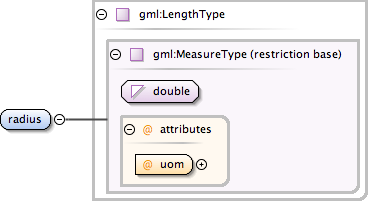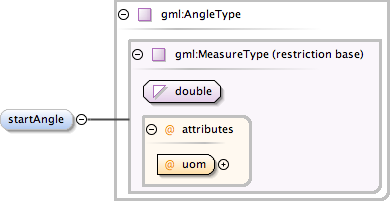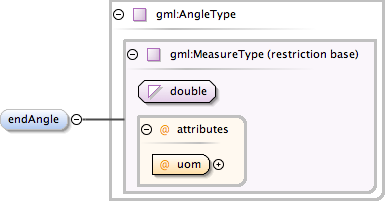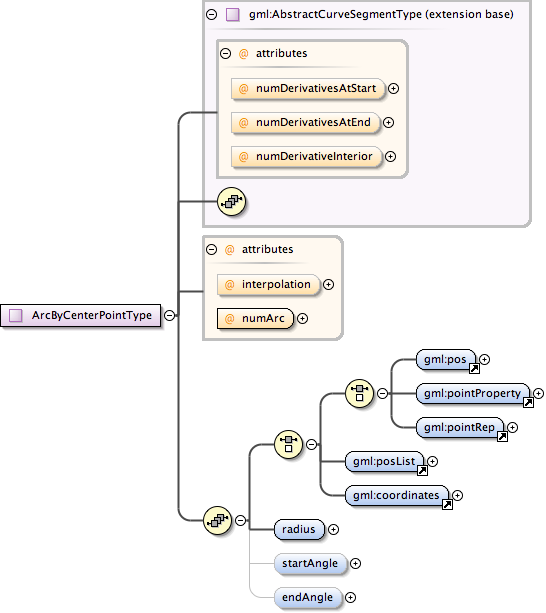### Showing:

 Annotations Attributes Diagrams Facets Properties Source Used by
Namespace http://www.opengis.net/gml
Annotations
 `The radius of the arc.`
DiagramType gml:LengthType
Type hierarchy
Properties
 content: complex
Attributes
QName Type Fixed Default Use Annotation
uom anyURI required
Source
 ``` The radius of the arc. ```
Schema location http://schemas.opengis.net/gml/3.1.1/base/geometryPrimitives.xsd
Element gml:ArcByCenterPointType / gml:startAngle
Namespace http://www.opengis.net/gml
Annotations
 `The bearing of the arc at the start.`
DiagramType gml:AngleType
Type hierarchy
Properties
 content: complex minOccurs: 0
Attributes
QName Type Fixed Default Use Annotation
uom anyURI required
Source
 ``` The bearing of the arc at the start. ```
Schema location http://schemas.opengis.net/gml/3.1.1/base/geometryPrimitives.xsd
Element gml:ArcByCenterPointType / gml:endAngle
Namespace http://www.opengis.net/gml
Annotations
 `The bearing of the arc at the end.`
DiagramType gml:AngleType
Type hierarchy
Properties
 content: complex minOccurs: 0
Attributes
QName Type Fixed Default Use Annotation
uom anyURI required
Source
 ``` The bearing of the arc at the end. ```
Schema location http://schemas.opengis.net/gml/3.1.1/base/geometryPrimitives.xsd
Complex Type gml:ArcByCenterPointType
Namespace http://www.opengis.net/gml
Annotations
 `This variant of the arc requires that the points on the arc have to be computed instead of storing the coordinates directly. The control point is the center point of the arc plus the radius and the bearing at start and end. This represenation can be used only in 2D.`
DiagramType extension of gml:AbstractCurveSegmentType
Type hierarchy
Used by
 Element gml:ArcByCenterPoint Complex Type gml:CircleByCenterPointType
Model (gml:pos | gml:pointProperty | gml:pointRep | gml:posList | gml:coordinates) , gml:radius , gml:startAngle{0,1} , gml:endAngle{0,1}
Children gml:coordinates, gml:endAngle, gml:pointProperty, gml:pointRep, gml:pos, gml:posList, gml:radius, gml:startAngle
Attributes
QName Type Fixed Default Use Annotation
 ```The attribute "interpolation" specifies the curve interpolation mechanism used for this segment. This mechanism uses the control points and control parameters to determine the position of this curve segment. For an ArcByCenterPoint the interpolation is fixed as "circularArcCenterPointWithRadius".```
numArc integer 1 required
 `Since this type describes always a single arc, the attribute is fixed to "1".`
numDerivativeInterior integer 0 optional
 ```The attribute "numDerivativesInterior" specifies the type of continuity that is guaranteed interior to the curve. The default value of "0" means simple continuity, which is a mandatory minimum level of continuity. This level is referred to as "C 0 " in mathematical texts. A value of 1 means that the function and its first derivative are continuous at the appropriate end point: "C 1 " continuity. A value of "n" for any integer means the function and its first n derivatives are continuous: "C n " continuity. NOTE: Use of these values is only appropriate when the basic curve definition is an underdetermined system. For example, line string segments cannot support continuity above C 0 , since there is no spare control parameter to adjust the incoming angle at the end points of the segment. Spline functions on the other hand often have extra degrees of freedom on end segments that allow them to adjust the values of the derivatives to support C 1 or higher continuity.```
numDerivativesAtEnd integer 0 optional
 ```The attribute "numDerivativesAtEnd" specifies the type of continuity between this curve segment and its successor. If this is the last curve segment in the curve, one of these values, as appropriate, is ignored. The default value of "0" means simple continuity, which is a mandatory minimum level of continuity. This level is referred to as "C 0 " in mathematical texts. A value of 1 means that the function and its first derivative are continuous at the appropriate end point: "C 1 " continuity. A value of "n" for any integer means the function and its first n derivatives are continuous: "C n " continuity. NOTE: Use of these values is only appropriate when the basic curve definition is an underdetermined system. For example, line string segments cannot support continuity above C 0 , since there is no spare control parameter to adjust the incoming angle at the end points of the segment. Spline functions on the other hand often have extra degrees of freedom on end segments that allow them to adjust the values of the derivatives to support C 1 or higher continuity.```
numDerivativesAtStart integer 0 optional
 ```The attribute "numDerivativesAtStart" specifies the type of continuity between this curve segment and its predecessor. If this is the first curve segment in the curve, one of these values, as appropriate, is ignored. The default value of "0" means simple continuity, which is a mandatory minimum level of continuity. This level is referred to as "C 0 " in mathematical texts. A value of 1 means that the function and its first derivative are continuous at the appropriate end point: "C 1 " continuity. A value of "n" for any integer means the function and its first n derivatives are continuous: "C n " continuity. NOTE: Use of these values is only appropriate when the basic curve definition is an underdetermined system. For example, line string segments cannot support continuity above C 0 , since there is no spare control parameter to adjust the incoming angle at the end points of the segment. Spline functions on the other hand often have extra degrees of freedom on end segments that allow them to adjust the values of the derivatives to support C 1 or higher continuity.```
Source
 ``` This variant of the arc requires that the points on the arc have to be computed instead of storing the coordinates directly. The control point is the center point of the arc plus the radius and the bearing at start and end. This represenation can be used only in 2D. GML supports two different ways to specify the control points of a curve segment. 1. A "pos" (DirectPositionType) or "pointProperty" (PointPropertyType) element. The "pos" element contains a center point that is only part of this curve segment, a "pointProperty" element contains a point that may be referenced from other geometry elements or reference another point defined outside of this curve segment (reuse of existing points). 2. The "posList" element can be used to specifiy the coordinates of the center point, too. The number of direct positions in the list must be one. Deprecated with GML version 3.1.0. Use "pointProperty" instead. Included for backwards compatibility with GML 3.0.0. Deprecated with GML version 3.1.0. Use "posList" instead. The radius of the arc. The bearing of the arc at the start. The bearing of the arc at the end. The attribute "interpolation" specifies the curve interpolation mechanism used for this segment. This mechanism uses the control points and control parameters to determine the position of this curve segment. For an ArcByCenterPoint the interpolation is fixed as "circularArcCenterPointWithRadius". Since this type describes always a single arc, the attribute is fixed to "1". ```
Schema location http://schemas.opengis.net/gml/3.1.1/base/geometryPrimitives.xsd
Attribute gml:ArcByCenterPointType / @interpolation
Namespace No namespace
Annotations
 ```The attribute "interpolation" specifies the curve interpolation mechanism used for this segment. This mechanism uses the control points and control parameters to determine the position of this curve segment. For an ArcByCenterPoint the interpolation is fixed as "circularArcCenterPointWithRadius".```
Type gml:CurveInterpolationType
Properties
Facets
 enumeration linear enumeration geodesic enumeration circularArc3Points enumeration circularArc2PointWithBulge enumeration circularArcCenterPointWithRadius enumeration elliptical enumeration clothoid enumeration conic enumeration polynomialSpline enumeration cubicSpline enumeration rationalSpline
Used by
 Complex Type gml:ArcByCenterPointType
Source
 ``` The attribute "interpolation" specifies the curve interpolation mechanism used for this segment. This mechanism uses the control points and control parameters to determine the position of this curve segment. For an ArcByCenterPoint the interpolation is fixed as "circularArcCenterPointWithRadius". ```
Schema location http://schemas.opengis.net/gml/3.1.1/base/geometryPrimitives.xsd
Attribute gml:ArcByCenterPointType / @numArc
Namespace No namespace
Annotations
 `Since this type describes always a single arc, the attribute is fixed to "1".`
Type integer
Properties
 use: required fixed: 1
Used by
 Complex Type gml:ArcByCenterPointType
Source
 ``` Since this type describes always a single arc, the attribute is fixed to "1". ```
Schema location http://schemas.opengis.net/gml/3.1.1/base/geometryPrimitives.xsd